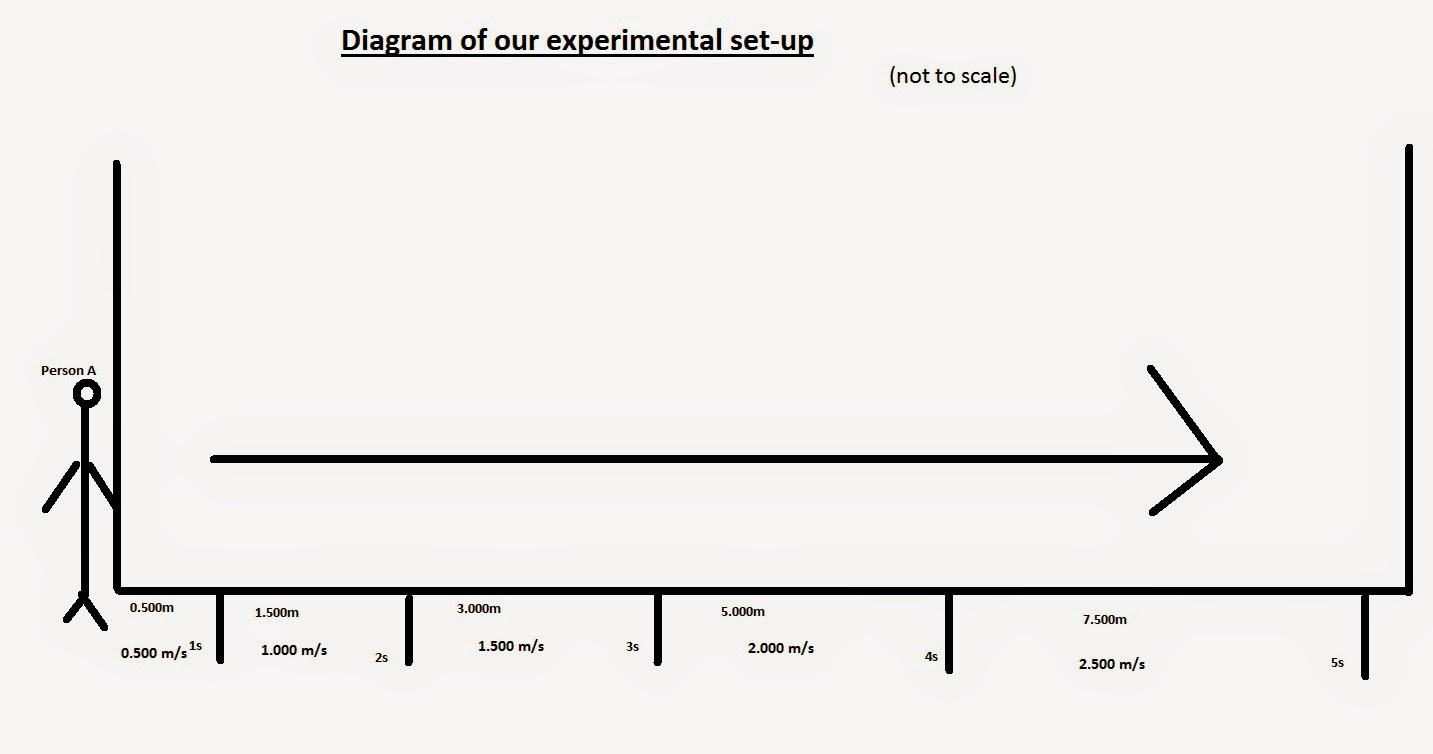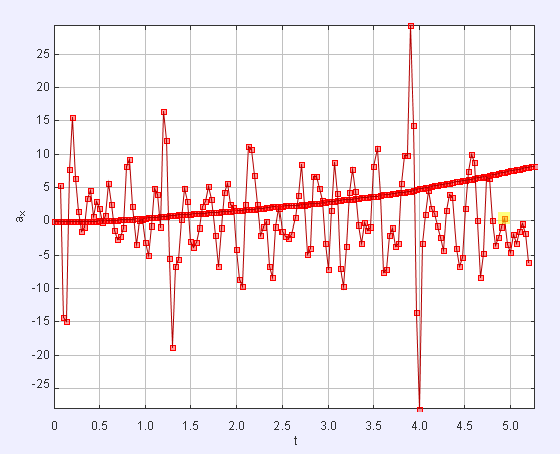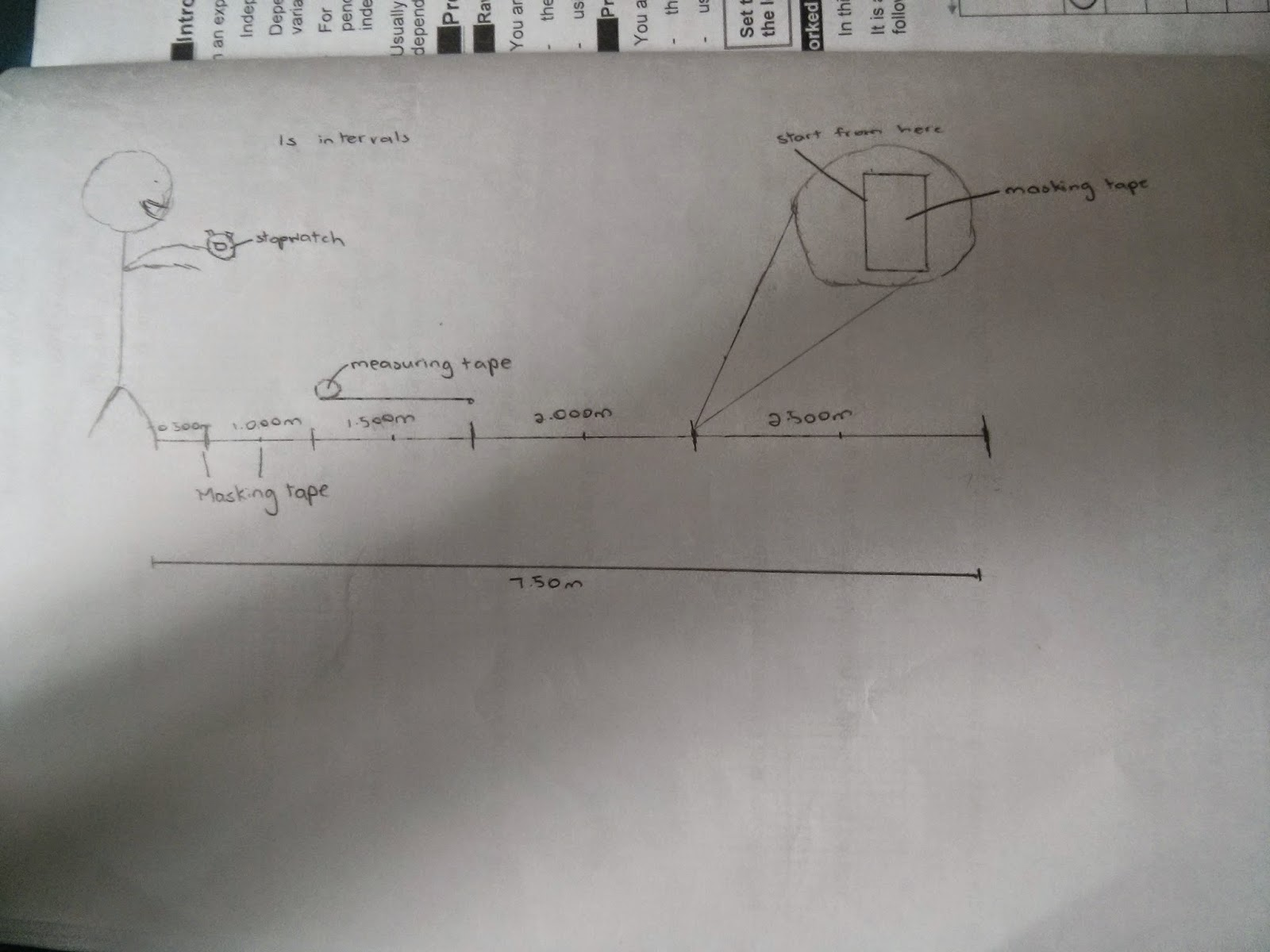##(our rough planning on paper) Hypothesis: the displacement time graph would be in an increasingly increasing graph. The velocity time graph would be increasing constantly. The acceleration time graph would be a line with a gradient of 0For the first second, the velocity is 0.50 m/s thus the person travels 0.50 m. For the second second, since acceleration is 0.50 m s^2, the velocity increases by 0.50 m/s to 1.00 m/s. The person then walks 1.000 m to a displacement of 1.50 m and so on. That is our calculations. Results Displacement time graphValue of acceleration:0.3542 m s^-2 Velocity time graphValue of acceleration: 0.4500 m s^-2 Acceleration time graphValue of acceleration: 0.4011 m s^-2 Evaluation and conclusion The results have not been quite close. The value of acceleration calculated from the best fit curve in displacement-time graph is 0.3542 m s^-2, far differing from the actual value of 0.50. It may be due to these sources of error such as random error due to human reaction time. For instance, we cannot reach each marking very accurately within each second as we have to look at the stopwatch also. Also, it may be due to wrong calculation. Our method theoretically makes the v-t graph like a stair-shape, instead of a smooth increasing line with a gradient of 0.50. This will definitely affect the a-t graph as the distance we travel is not actually the same. The other graphs' value of acceleration are least accurate from the S-t graph as many information of the actual values are gone when tracker decides to convert S-t graph to v-t and a-t graph.

### Kah Leong, Yi Meng, Daven

We are supposed to conduct an experiment to take a video of a person walking at a constant acceleration of 0.5 m/s^2 for 5 seconds.
For this, we started off at a original length of 0.500m, afterwards we increased the interval by 0.500m. By traveling the new marked distance in 1 second, we will be able to get the acceleration of 0.5m/s^2.

Our setup:

We expect our displacement time graph to be a curved line as we cover more distance within the same period of time (1 second)

Displacement-time graph:
From our s-t graph, our acceleration is 0.54ms^-2.

For the acceleration time graph, it was really hard to see the best fit line as the points are relatively packed together, thus we zoomed in and changed the y axis to 0.00-1.00 and we found out that our acceleration is around 0.375 m/s^2.
Acceleration-time graph

From the graph, the Tracker software uses the formula V=u+at to form this graph, but presents it in the form of v=at +b. Therefore, we know that our value of acceleration will be the value of "a" that is give in Tracker, which is 0.5560 ms^-2.

Since data is lost when tracker converts the graph from st to tv and finally to at (by comparing them with the previous points) , the most reliable acceleration value will be from our st graph. Therefore, our acceleration that we got in this activity is 0.54 ms^-2.

Why is it not a constant acceleration and not 0.5m/s^2?
It is very difficult to ensure that the acceleration is the same between each step. Also, instead of maintaining a constant rate of acceleration, we only increased our speed at different moments or intervals, hence the acceleration was not constant. This might also result in a difference in the distance covered within one second when compared to our proposed length.
Secondly, another source of error will be that It is almost impossible for us to reach our marked interval at exactly 1.00 seconds. We might reach the marking slightly early or slightly later and this might affect the best fit lines of both graphs.

As for the assumptions, we assume that all our movement  will be accurately tracked by the software.
the area covered by our hair in the video is relatively large. The software can track any point of the hair as it will still be the same as  the mass point that we have identified. This might result in a difference in the distance covered within one second when compared to our proposed length.Acceleration against timeDisplacement against timePlanning

Actual plan:
We marked out intervals of 0.300m, 1.000m, 1.500m, 2.000m,2.5000m on the ground using masking tape, measured using a measuring tape. The markings on the ground represented the position of each step we had to take to achieve a constant acceleration of 0.50m/s^2. Using a stopwatch, we then timed ourselves to walk through the distance.

Evaluation of results and design:
Using a software tracker, we plotted a graph. From the graph, we can conclude that the displacement and acceleration increases as the time increases.
We assumed that the video was taken at the same position throughout. A source of error might be that the software tracker had inaccurately tracked the movement of the person walking and might be affected disruptions in the video. Also, random error such as human action time might be present when timing using the stopwatch.

The acceleration was not at a constant 0.5ms^-2, contrary to expectations, due to the form of human locomotion, in which it is very difficult to ensure that the acceleration is the same between each step. In addition, instead of maintaining a constant rate of acceleration, we only increased our speed at intervals, hence contributing to the inconsistency.